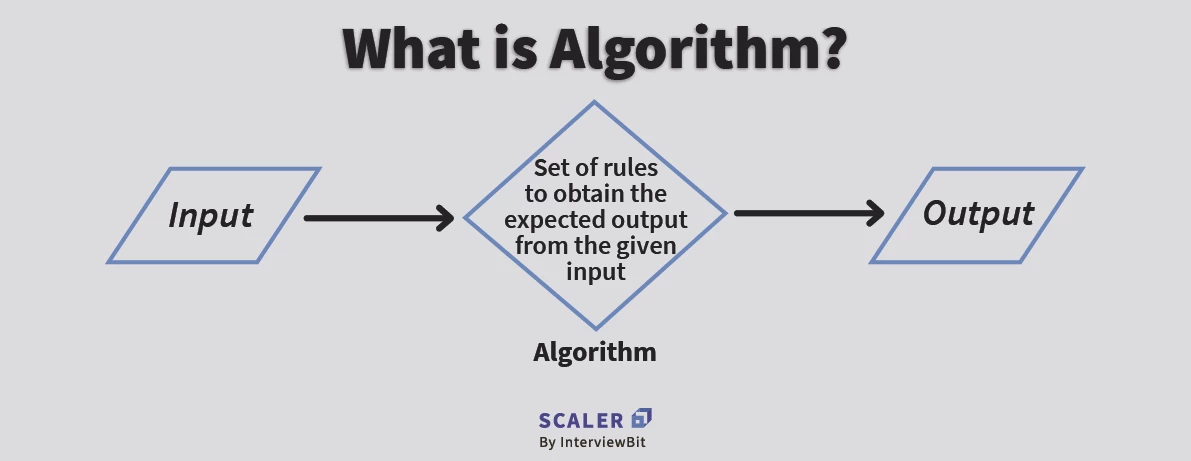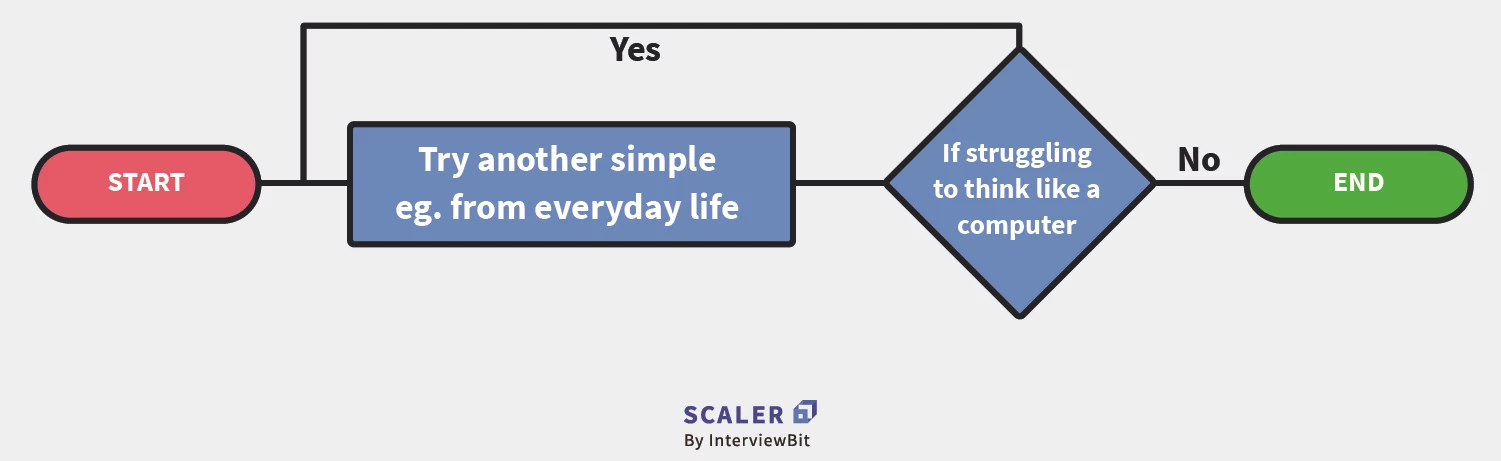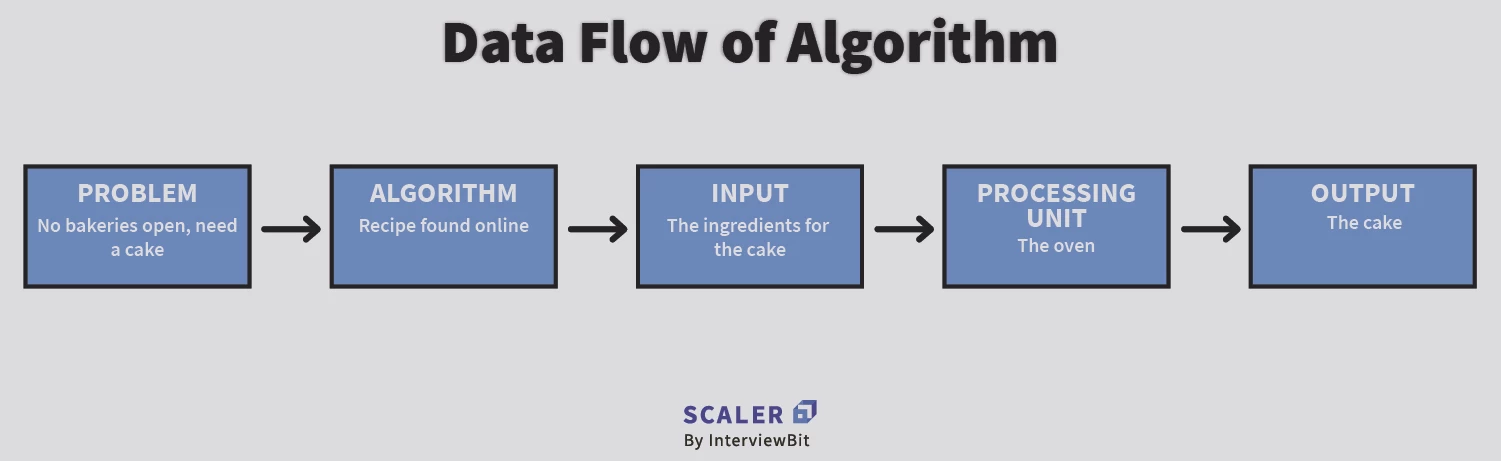# What Are Data Structures and Algorithms?

Challenge Inside! : Find out where you stand! Try quiz, solve problems & win rewards!
Learn via video courseDSA Problem Solving for Interviews using Java
By Jitender PuniaFree
4.9Enrolled: 1000DSA Problem Solving for Interviews using Java
Jitender PuniaFree
4.9
Enrolled: 1000

## Overview

More precisely, a data structure is a collection of data values, the relationships among them, and the functions or operations that can be applied to the data

Technically speaking, an algorithm is defined as a set of rules or a step-by-step procedure that are to be executed in a specific order to get the desired output.The formal definition of an algorithm is a finite set of steps carried out in a specific time for specific problem-solving operations, especially by a Computer.

Algorithms are independent of programming languages and are usually represented by using flowcharts or pseudocode.

## Characteristics of an Algorithm

A fact to be noted is that not all sets of instructions or procedures are an algorithm. A set of instructions should have all the relevant characteristics to qualify as an algorithm.

### The characteristics of algorithm are as follows-• Unambiguous– An algorithm should be clear and simple in nature and lead to a meaningful outcome i.e. they should be unambiguous.
• Input– It should have some input values.
• Output– Every algorithm should have well-defined outputs
• Finiteness– The steps of an algorithm should be countable and it should terminate after the specified number of steps.
• Effectiveness– Each step of the algorithm should be effective and efficient enough to produce results. The effectiveness of an algorithm can be evaluated with the help of two important parameters-
• Time Complexity-It is nothing but the amount of time taken by the computer to run the algorithm. We can also call it the computational complexity of an algorithm. It can either be best-case, average-case or worst-case. We always aim for the best-case for effectiveness.
• Space Complexity-It refers to the amount of computational memory needed to solve an instance of the problem statement.The lower the space complexity of an algorithm, the faster the algorithm will work.
• Language Independent– Algorithms should be language independent. In other words, the algorithm should work for all programming languages and give the same output.

## Data Flow of an Algorith• Problem- The problem can be any real world or a programmable problem. The problem statement usually gives the programmer an idea of the issue at hand, the available resources and the motivation to come with a plan to solve it.
• Algorithm- After analysing the problem, the programmer designs the step by step procedure to solve the problem efficiently. This procedure is the algorithm.
• Input- The algorithm is designed and the relevant inputs are supplied.
• Processing Unit- The processing unit receives these inputs and processes them as per the designed algorithm
• Output- Finally, after the processing is complete, we receive the favourable output of our problem statement.

## Why do we Need Algorithms?

Primarily, we need algorithms for the following two reasons-

• Scalability– When we have big real-world problems, we cannot tackle them on the macro level. We need to break them down into smaller steps so that the problem can be analyzed easily. Thus, algorithms facilitate scalability
• Performance– It is never easy to break down big problems into smaller modules. But algorithms help us achieve this. They help us make the problem feasible and provide efficient performance driven solutions

## How to Write an Algorithm?A simple Google search would tell you that there is no one “right way” to bake a cake. There are countless recipes available online- devised and tested by bakers around the world. Likewise, there are no predefined standards on how to write an algorithm.

The way we write algorithms is heavily influenced by the problem statement and the resources available. The common construct that is widely followed in case of algorithms is the use of pseudocode.

## Factors of an Algorithm

While designing an algorithm, we must consider the following factors-

• Modularity- If a big problem can be easily broken down into smaller ones, it facilitates modularity.
• Correctness- The analysis of the problem statement and consequently the algorithm should be correct. The algorithm should also work correctly for all possible test cases of the problem at hand.
• Maintainability- The algorithm should be designed in such a way that it should be easy to maintain and if we wish to refine it at some point.
• Functionality- The steps of an algorithm should successfully solve a real world problem.
• User-friendly- It should be easily understood by programmers.
• Simplicity- It should be the simplest possible solution to a problem. By simplicity, we refer to the fact that the algorithm should have the best-case time complexity.
• Extensible- It should be extensible i.e. the algorithm should facilitate reusability.

## Example

Example 1: Design an algorithm to accept three numbers and print their sum.

Step 1-START

Step 2- Declare variables x,y,z and sum

Step 3- Read the value of x,y and z

Step 4- Add x,y and z

Step 5-Store the output of Step 4 in sum

Step 6-Print sum

Step 7- STOP

Example 2: Design an algorithm to find the factorial of a number and print it.

Step 1-START

Step 2- Declare variables fact, num and i

Step 3- Initialize variables as: fact->1 and i->1

Step 4- Read the value of num

Step 5-Repeat the steps until num=i

• 5.1- fact->fact*i
• 5.2- i->i+1

Step 6- Print fact

Step 7- STOP

## Importance of Algorithms

The importance of algorithms can be classified as-

• Theoretical Importance- The best way to deal with any real-world problem is to break them down into smaller modules.The theoretical knowledge from pre-existing algorithms often help us to do so.
• Practical Importance- Just designing an algorithm theoretically or using some aspects of pre-existing ones is not enough. The real-world problems can only be considered to be solved if we manage to get practical results from it

Hence, an algorithm can be said to have both theoretical and practical importance.

## Cheatsheet for Algorithm In Data Structure

### Types of Algorithms

An algorithm is a sequential series of steps and processes for solving a problem. While there can be different types of algorithms for solving different problems, we consider the following algorithms to be important in programming.

As a starting point, here are the types of Algorithms

### 1. Linear Search

The linear search algorithm is also known as a sequential search algorithm and it is the simplest of all search algorithms. The linear search involves traversing the list completely and matching each element with the item whose location you are looking for. An item's location is returned if a match is found; otherwise, NULL is returned.

### 2. Binary Search

A binary search is a search algorithm that divides the search interval in half repeatedly to search a sorted array. Divide and conquer is the underlying principle of a binary search. It is imperative that the data collection should be sorted in order for this algorithm to work properly.

A binary search compares the middlemost item of a collection in order to find a particular item. An index of the item is returned if there is a match. The item is then looked up in the sub-array to the left of the middle item if the middle item exceeds the item. The item is otherwise searched for in the sub-array to the right of the middle item. The same process is repeated on the sub-array until the subarray is reduced to zero.

## Sorting Algorithms

### 1. Bubble Sort

In Bubble Sort, an element is swapped repeatedly until it is in the sorted or intended order. The movement of array elements is similar to the movement of air bubbles in water, which is why it is called bubble sort. Similar to bubbles in water rising to the surface, array elements in bubble sort move to the end each time they are iterated.

### 2. Insertion Sort

Insertion sort involves placing an unsorted item at its proper place in each iteration of a sorting algorithm. It is similar to how we sort the cards in our hands in a card game.

First, we select an unsorted card, assuming that the first card is already sorted. The unsorted card should be put on the right if it is greater than the card in hand, otherwise, it should be placed on the left. A similar process is followed in sorting other unsorted cards.

### 3. Quick sort

The Quicksort algorithm involves divide-and-conquer. In this method, one element is selected as the pivot, and the others are divided into two sub-arrays based on whether they are less or greater than the pivot. This method is therefore sometimes referred to as partition-exchange sort. This can be done in place, with only a little amount of additional RAM required for sorting..

### 4. Selection Sort

Despite its simplicity and straight forward nature, selection sort is a powerful sorting algorithm. By comparing the items in place, selection-based sorting divides the list into two sections, left and right of the sorted items. There is no data in the sorted section, while all the data is in the unsorted section. A small list can be sorted using selection sort.

### 5. Merge sort

Basically, merge sort splits the input into two halves, repeats the process on both halves, and merges the sorted halves together. From top to bottom, the algorithm divides the list into progressively smaller pieces until only the individual elements remain. It then moves back up to ensure that the merged lists are sorted.

### 6. Counting Sort

Using this algorithm, elements will be sorted by the number of times each element occurs. It is a fast and reliable method to sort data. The algorithm calculates how many elements are associated with each unique value. Afterward, this data is sorted according to specific mathematical calculations.

Radix sort is a sorting algorithm that groups the digits of the same place value together before sorting the elements. Following that, sort the elements in order of increasing/decreasing value.

Let's say we have an array of 6 elements. As a first step, we will sort the elements by place value. Our next step will be to sort elements according to the tenth place. The process continues until the last significant place is reached.

### 8. Bucket Sort

Using bucket sort, the elements of an array are divided into several groups called buckets. The buckets are then sorted by applying any of the available sorting algorithms or by recursively applying the same bucket algorithm. The sorted buckets are then concatenated to create a final sorted array.

### 9. Shell Sort

The sorting algorithm is an extended version of insertion sort; it sorts the elements that are far apart at first, then it reduces the distance between them at the end. The gap between elements is called an interval. Knuth's formula can be used to calculate this interval.

### 10. Heap Sort

The binary heap data structure is used for heap sorting, which is a comparison-based sorting technique . In heap sort, each element in the list is eliminated from the heap part of the list, then it is inserted into the sorted part of the list.

## Recursive Algorithm

Recursion is the basis for this kind of algorithm. Using recursion, a problem is broken into sub-problems and calls itself repeatedly until an underlying condition is able to solve it.

## Divide And Conquer Algorithm

The divide and conquer algorithm divides the problem into two sections, the first section dividing it into subproblems of the same type. Section two involves solving smaller problems independently, combining the results, and calculating the final answer.

## Dynamic Programming Algorithms

An algorithm of this type is also referred to as a memoization algorithm because it stores the previously calculated result in order to avoid recalculating it. In dynamic programming, we divide complex problems into smaller overlapping subproblems and store the results for later use in Dynamic Programming.

## Greedy Algorithm

A greedy algorithm builds solution part by part. In order to decide which part to proceed to next, we look at the benefit it provides immediately. Previous decisions are never taken into account.

## Backtracking Algorithm

By using backtracking algorithm, problems are solved incrementally, i.e. they are solved recursively by adding pieces one at a time, and removing the solutions that do not meet the constraints of the problem at any point in time.

## Hashing Algorithm

A hashing algorithm operates the same as a searching algorithm, but it includes an index with an ID. We assign a key to specific data when we hash it.

## Issues While Working on Algorithms

The most common issue that we face while working on algorithms is- “How do I design it?”

Not all problems will have an easy solution and along with that, we need the solutions to be efficient as well. Sometimes, this may cause the programmers to feel stuck.

## Conclusion

By now, you have successfully gathered a clear idea about-

• What is an algorithm?
• Characteristics of an algorithm
• The how and why of an algorithm
• The importance and issues
• Some easy to understand examples

The next time you do a task now, you will try to relate it with an algorithm. So start exploring and keep an eye out for learning more!